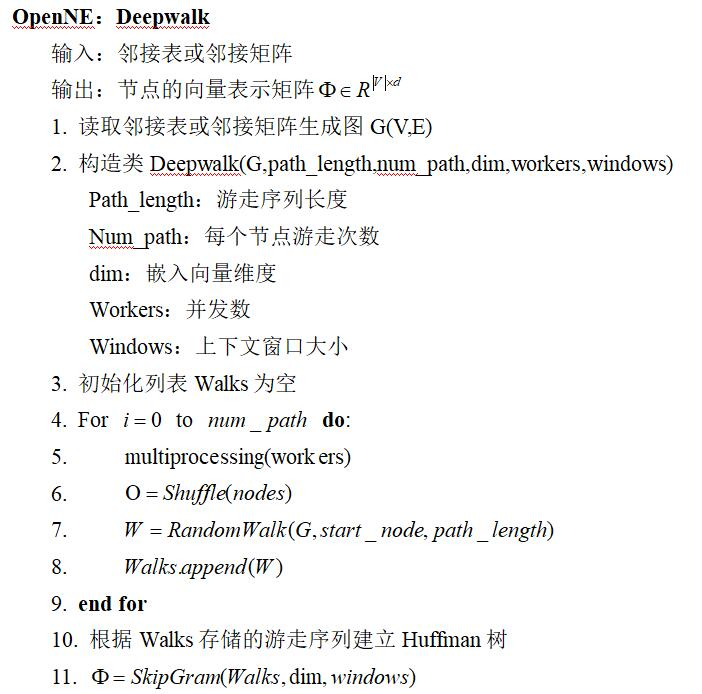# Deepwalk算法原理深度研究

## 网络嵌入

Posted by Yunlongs on April 26, 2019

# Deepwalk算法原理深度研究

## 3. 算法设计

$W_{1}^{n}=\left(w_{0}, w_{1}, \ldots, w_{n}\right)$

$w_i$代表着此句子中的第i个单词，我们的目标是使在给定前n-1个词的情况下，出现$w_n$的概率最大，即最大化参数使得$\operatorname{Pr}\left(w_{n} | w_{0}, w_{1}, \cdots, w_{n-1}\right)$取最大值。

$V_{1}^{N}=\left(v_{0}, v_{1}, \ldots, v_{n}\right)$

$\operatorname{Pr}\left(v_{i} \|\left(v_{1}, v_{2}, \ldots, v_{i-1}\right)\right)$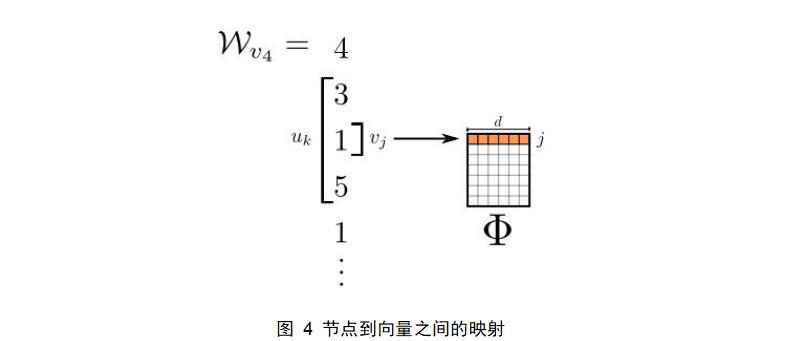$\operatorname{Pr}\left(v_{i} \|\left(\Phi\left(v_{1}\right), \Phi\left(v_{2}\right), \ldots, \Phi\left(v_{i-1}\right)\right)\right)$

（1）不再使用上下文来预测一个缺失词，而是使用缺失词来预测上下文。（2）上下文由目标词的左右两侧组成，不仅仅是一边。（3）最大化词在上下文中出现的概率，并且不需要知道对于给定词的相对偏移位置。新的优化目标总结如下： $\log \operatorname{Pr}\left(\left\{v_{i-w}, \ldots, v_{i-1}, v_{i+1}, \ldots, v_{i+w}\right\} \| \Phi\left(v_{i}\right)\right)$

## 4. 嵌入向量产生过程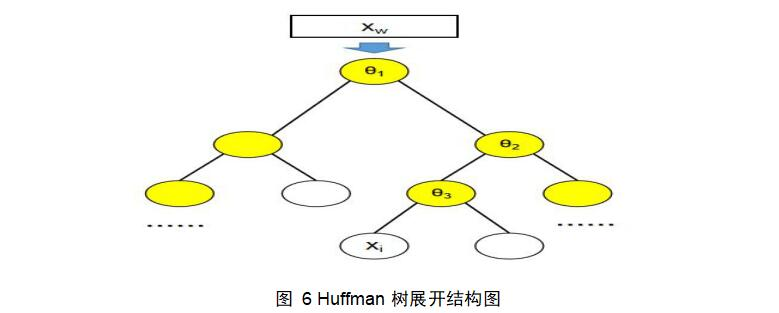根据图6的Huffman树，还需要定义以下符号：（1）$p^w$为从根节点出发到达词w所对应叶子节点的路径；（2）$l^w$为$p^w$上所包含的节点个数；（3）${d_j}^w$代表着$p^w$中第j个节点对应的编码，左节点编码为0，右节点编码为1。 现在继续考虑之前的优化目标（1.1），根据乘法公式，可以将其展开为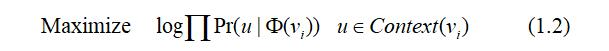将网络节点替换为图6中所使用的词向量后，优化目标就变成了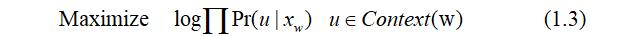基于Hierarchical Softmax的Skip-gram模型中是这样来表示在已知中心词$x^w$求其上下文词的概率，即$\operatorname{Pr}\left(u | x_{w}\right)$：以图6为例，从中心词$x^w$所对应的根节点开始形成到一叶节点$x_i$的路径$p^i$，在$p^i$中从根节点开始每遇到一个非叶节点就要做一次二分类，使用逻辑回归分别计算出到该非叶节点2个孩子的概率，规定该飞叶子节点到其左边孩子节点的概率为$1-\sigma\left(x_{w}^{T} \theta\right)$，到其右边孩子节点的概率为$\sigma\left(x_{w}^{T} \theta\right)$，将$p^i$所有经过节点的概率相乘，就得到了$\operatorname{Pr}\left(x_{i} | x_{w}\right)$。 但是这里得到的是$\operatorname{Pr}\left(x_{i} | x_{w}\right)$，$x_i$只是语料中心词w上下文的一个，若我们对上下文所有词都进行这样的计算，同时将目标函数（1.3）进行最大化，再经过softmax归一化后得到的将是语料中每个词成为中心词w上下文的概率，概率最大的2w个词即可作为预测出的上下文。 但是我们的任务是获得词向量，研究目标函数的优化过程可以发现，所需的词向量其实只是优化过程中产生的一个副产品，所以为了获得词向量并不需要完整的Skip-gram模型，优化过程如下：对目标函数(1.3)使用随机梯度上升算法进行最大化，可以得到参数xw和θ的更新公式为：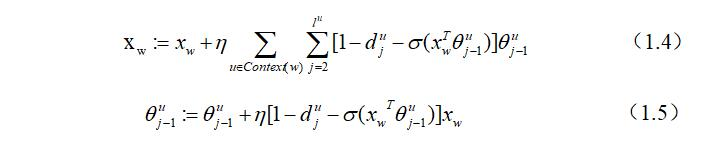由此可得基于Hierarchical Softmax的Skip-gram模型运作流程总结如下：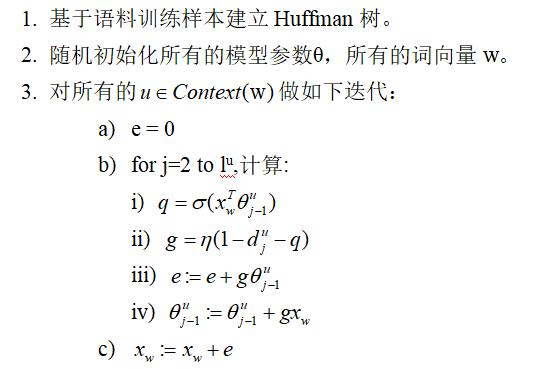这是根据文献【1】中词嵌入技术发明者提出skip-gram模型的理论实现方案，由流程中的c)可得该模型运行一次仅能得出中心词w的词向量，嵌入效率低于能一次更新所有上下文词向量的CBOW模型。在之后的word2vec技术实现中，根据上下文的作用是相互的，期望最大化$\operatorname{Pr}\left(u | x_{w}\right)$等同于期望最大化$\operatorname{Pr}\left(x_{w} | u\right)$，因此将优化目标由变成了$\operatorname{Pr}\left(x_{w} | u\right)$。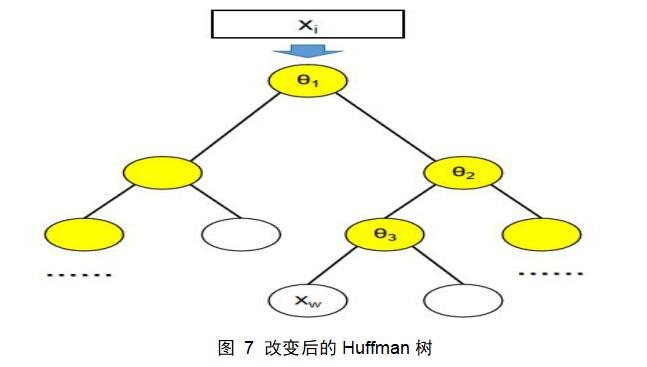如图7所示，Huffman树的输入变成了上下文中的词的向量xi，目标路径通往中心词w对应的节点。优化后的基于Hierarchical Softmax的Skip-gram模型运作流程如下：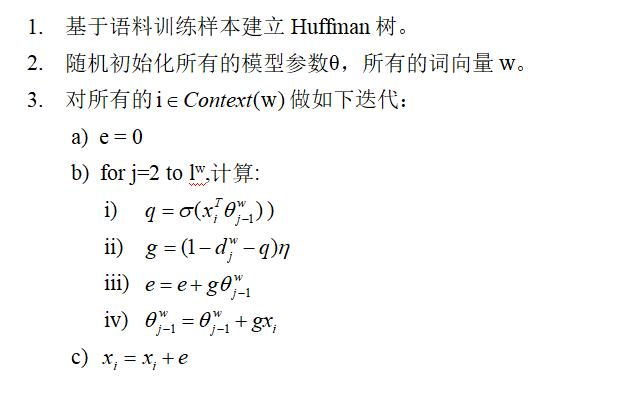## 5. Deepwalk算法流程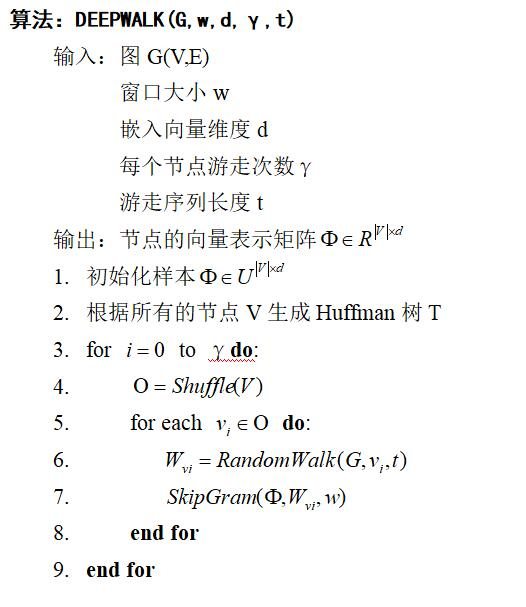## 6. OpenNE中Deepwalk算法实现流程